Predicting the survival time for diffuse large B-cell lymphoma using microarray data | OMICS International
Journal of Molecular and Genetic Medicine

# Predicting the survival time for diffuse large B-cell lymphoma using microarray data

Mehri Khoshhali1, Hossein Mahjub2,*, Massoud Saidijam3, Jalal Poorolajal2, Ali Reza Soltanian2

1Department of Biostatistics & Epidemiology, School of Public Health, Hamadan University of Medical Sciences, Hamadan, Iran,

2Research Center for Health Sciences, Department of Epidemiology & Biostatistics, School of Public Health, Hamadan University of Medical Sciences, Hamadan, Iran,

3Research Center for Molecular Medicine, Department of Molecular Medicine and Genetics, School of Medicine, Hamadan University of Medical Sciences, Hamadan, Iran

*Corresponding Author:
Hossein Mahjub
Tel: +98 811 8260661
Fax: +98 811 8255301
Email: [email protected]

Received date: 08 September 2011; Revised date: 26 April 2012; Accepted date: 30 April 2012; Published date: 23 May 2012

© Copyright The Authors: Published by Library Publishing Media. This is an open access article, published under the terms of the Creative Commons Attribution Non-Commercial License (http://creativecommons.org/licenses/by-nc/2.5). This license permits non-commercial use, distribution and reproduction of the article, provided the original work is appropriately acknowledged with correct citation details.

Visit for more related articles at Journal of Molecular and Genetic Medicine

#### Keywords

Lymphoma, gene expression, microarray, survival analysis, dimension reduction, ridge regression

#### Introduction

Cancers of immune system are classified into two major groups including Hodgkin’s lymphoma (HL) and non- Hodgkin’s lymphoma (NHL). B-cell NHL lymphomas comprise a large group of lymphomas, such as, Burkitt lymphoma, diffuse large B-cell lymphoma (DLBCL), follicular lymphoma, immunoblastic large cell lymphoma, precursor B-lymphoblastic lymphom, and mantle cell lymphoma. T-cell NHL lymphomas include mycosis fungoides, anaplastic large cell lymphoma and precursor T-lymphoblastic lymphoma (Cheng and Walkom, 2008).

In the USA, NHL is the fifth prominent position of new cancer cases among men and women. In 2002, 2.8% of all cancers were NHL worldwide. NHLs are more common in the developed countries (Cheng and Walkom, 2008). DLBCL, the most common subset of NHL, is clinically heterogeneous so that only 40% of patients respond to current treatments and have prolonged survival, while the remainders are submitted against the disease. Using microarray technology, systematic patterns of gene expression are examined in B-cell malignancies (Alizadeh et al, 2000). In order to study the behavior and function of cells, it is possible to investigate the expression levels of thousands of genes simultaneously using microarray technology (Ho et al, 2006). Different levels of gene expression in DLBCL lead to differences in tumor proliferation rate, host response and the different situation of tumor (Alizadeh et al, 2000). Accordingly, the survival time of cancerous patients can be estimated based on gene expression profile (Bovelstad et al, 2007).

The discovery of the relationship between survival time and tumor expression profiles provided the possibility to achieve more accurate diagnosis and more advanced treatment (Bovelstad et al, 2007). In survival analysis, the time to reach an event may sometimes be censored. In this case, using the standard statistical methods is not possible. Many methods are introduced for such data (Ma et al, 2006; Martinussen and Scheike, 2009). One of the most popular methods is Cox proportional hazard model. In the classic situation in which the number of sample n is larger than the number of variables p (i.e., n>p), the parameters of regression are estimated by maximizing Cox partial likelihood function, but in microarray data in which the number of sample n is smaller than the number of variables p (n<p), using this method alone may not be appropriate (Bovelstad et al, 2007).

In recent years, both simple dimension reduction methods and more complex methods have been widely used to predict the survival of cancer patients based on gene expression data (Bovelstad et al, 2007; Li and Li, 2004; Li, 2010). But few studies conducted to compare dimension reduction methods for this purpose (Bovelstad et al, 2007). The present study was conducted based on gene expression data using Cox regression in combination with seven dimension reduction methods in order to predict survival time in patients with DLBCL and to determine the influential genes on survival time.

#### Materials and Methods

In this historical cohort, which was performed in 2010, we used DLBCL dataset including 4026 genes expression obtained based on array method of complementary DNA (Alizadeh et al, 2000). Data are available from: http://llmpp. nih.gov. The dataset contained survival time of 40 DLBCL patients. The desired event was death due to DLBCL disease and response variable was survival time of DLBCL patients after chemotherapy. Almost 45% of the survival time of patients was right censored.

The expression of gene was not specified for a large part of the dataset because of missing data. Since, the statistical methods used in this study could not be applied to missing data, these genes were deleted. After deleting this part of the dataset, the number of remaining genes reduced to 2042 genes.

To predict survival of patients with DLBCL based on gene expression, we combined Cox regression model (1) with seven dimension reduction methods (Bovelstad et al, 2007). A popular survival hazard function is introduced by Cox in the form: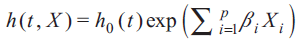(1)

where, Xi = X1, X2i, …, Xp represents predictive or explanatory variables (gene expression values), h0(t) represents basic or primary function of risk, and h(t) represents hazard rate or risk of death at time t.

Dimension reduction methods that used in this study were univariate selection (Bovelstad et al, 2007), forward stepwise selection (Bovelstad et al, 2007), principal component regression (Bovelstad et al, 2007), supervised principal component regression (Bair et al, 2006; Bovelstad et al, 2007), partial least squares regression (Bovelstad et al, 2007; Nygard et al, 2008), ridge regression and the Lasso (Bovelstad et al, 2007).

The dimension reduction methods need the tuning parameter to determine the dimension reduction or shrinkage rate. The tuning parameter for univariate and forward stepwise selection methods is the number of genes, for two principal component methods and partial least squares regression is the number of linear combinations, and for ridge regression and Lasso is the shrinkage rate. In this study, the optimal tuning parameter (λ) was determined by cross-validation method (Bovelstad et al, 2007). Dimension reduction and prediction methods are summarized as follows:

In the univariate selection method, each gene expression value was tested alone on survival time using the univariate Cox regression model. Following testing each gene, they were sorted according to their P values. Then, λ first arranged genes that had the lowest P value were entered in multiple Cox regression model (Bovelstad et al, 2007).

In the forward stepwise selection method, at the first stage of the study, the most significant gene was selected using univariate Cox regression. In the second step, the selected gene and the other most significant gene was added to the Cox regression method. This approach continued until λ genes were selected (Bovelstad et al, 2007).

In principal component regression (PCR), the principal component of gene expressions was formed. Then, λ first principal components that had the greatest variance were entered in Cox regression model (Bovelstad et al, 2007).

In supervised principal component regression method (SPCR), at first, the λ1 percent of the ranked genes were selected according to their P value using univariate Cox regression. Then a principal component regression method was applied on this subset of genes. Finally, λ2 principal components were entered in the multiple Cox regression model. In this method, the tuning parameter was two-state (λ=λ12) (Bair and Tibshirani, 2004; Bair et al, 2006; Bovelstad et al, 2007).

In partial least squares regression (PLS), PLS components were similar to the principal components except that the PLS used combinations that were correlated with survival time. There were many methods to perform PLS for Cox regression. We used the method which was proposed by Nygard et al (Bovelstad et al, 2007; Nygard et al, 2008).

In ridge regression, the regression coefficients were shrunk by imposing a penalty on the square value of the coefficients. For the Cox model, regression coefficients were estimated by maximizing the penalized log partial likelihood function: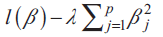where l(β) is log partial likelihood function and,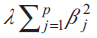was the penalty term (Bovelstad et al, 2007)

Also, lasso is a method for variable selection and shrinkage in Cox proportional hazard model. This method, similar to ridge regression, shrinks regression coefficients towards zero. The difference is that the penalty term is the absolute value of coefficients instead of squared values of the Cox regression coefficients (Bovelstad et al, 2007).

In order to evaluate the selected methods, dataset was split randomly into two parts, the training-set including a sample of 27 for estimation of the regression coefficients and the test-set including a sample of 13 for evaluating the performance of prediction model (Bovelstad et al, 2007). Data splitting was repeated 50 times randomly because if we used only one split of the data, it was not known to which extent the values of resulting criteria may depend on the actual training/test randomization (Bovelstad et al, 2007).

The estimation of parameters (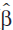train) for each dimension reduction method was obtained through the following two stages. First, the optimal tuning parameter value (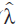train) was defined using the 10-fold cross-validation, then the specifiedtrain was estimated usingtrain. For each ith patient in the test set, prognostic index (PI) was estimated as follows (Bovelstad et al, 2007);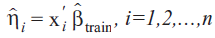(2)

where, xi was gene expression vector for ith patient in the test set.

The performance of a method was appropriate when the PI described the actual survival time of patients. There are different criteria to evaluate how well the survival times are described. The criteria which were examined in this study included log rank test, prognostic index test and deviance (Bovelstad et al, 2007).

In log rank test, patients in the test set were divided into two groups based on the median of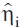the index. To evaluate the performance of this grouping, log rank test was applied. The P value was used as a basis for evaluation (Bovelstad et al, 2007).

Prognostic index is a criterion in which theindex is used as a continuous covariate in a Cox regression model. The P value of the likelihood ratio test was considered as basis for evaluation (Bovelstad et al, 2007).

Also, deviance is a criterion in which the difference in deviance between the fitted model and the null model is computed as 2[l(test) (train ) − l (test) (0)] , where l(test) (train ) and l(test) (0)] are the Cox log partial likelihood for the test data evaluated intrain train and zero, respectively. Its P value is used as a basis for evaluation (Bovelstad et al, 2007).

In these criteria, the comparison of the fitted model was done with the null model in which all PIs were zero and was equivalent to the model with no gene expression for prediction. Three criteria were calculated for each of the seven dimension reduction methods in 50 training/test split of data and the median of each criteria was considered as basis for evaluation. The smaller values of the criterion expressed the better performance of prediction (Bovelstad et al, 2007).

In order to determine the influential genes on survival time for PCR, SPCR, PLS and ridge regression methods, different cut points were tried. Cut point is an index for keeping or removing predictive variable according to its Cox regression coefficient. For standardized variables, the coefficients close to zero express a little effect on the outcome. Therefore, the variable can be excluded from the model. Different values of cut point resulted in different number of variables. The cut point value is determined based on sample size. MATLAB r2008a and RKWard statistical programs were used for data analysis.

#### Results

The survival time of the patients ranges from 1.3 to 129.9 months. The median survival time, using Kaplan–Meir approach, was 32.5 months. Figure 1 shows the box plots of P values resulted from three performance criteria for seven prediction methods in 50 training/test split of data. Accordingly, ridge regression method shows the smallest median among the three criteria and thus has highest capability of prediction. The spread of box plot diagrams represents difference between the results of one split to another.

Figure 1. Box plots of p-value resulting from three performance criteria for seven prediction methods. The horizontal lines represent the null model with no gene information included. The smaller the value of each criterion, the better the performance of prediction will be. Uni: Univariate selection, FS: Forward stepwise selection, PCR: Principal component regression, SPCR: Supervised PCR, PLS: Partial least square.

Ridge regression is a shrinkage method. Sixteen genes had absolute values greater than the cut point of 0.06 (Table 1). Finally, four out of 16 genes were selected using forward stepwise selection in last step using Cox regression model (P<0.05). Table 2 shows the coefficients, hazard ratios and their 95% confidence intervals for these four genes. According to the estimated prognostic (PI) index and the three mentioned criteria, these four genes had the highest capability for prediction (P<0.001). Based on calculated hazard ratio, four genes were diagnosed as influential factors on DLBCL survival. The expression of genes GENE3555X and GENE3807X decreased the survival time (P=0.008 and P=0.003, respectively), whereas the expression of genes GENE3228X and GENE1551X increased survival time (P=0.002 and P<0.001, respectively).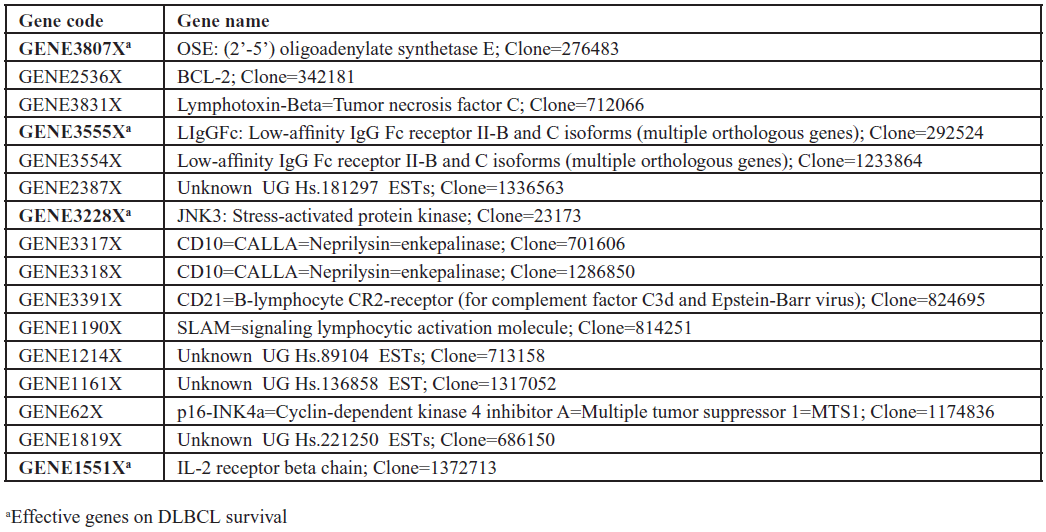Table 1. Influential genes on DLBCL survival based on the cut point value of 0.06 using ridge regression model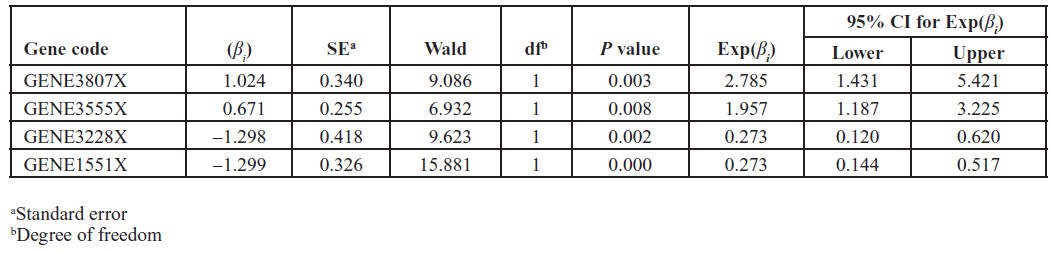Table 2. Estimated parameters using Cox regression model

#### Discussion

It was indicated that, based on three mentioned criteria, ridge regression had higher capability of prediction than other dimension reduction methods. Based on the pre- determined cut point for ridge regression coefficients and using forward stepwise selection of Cox survival model, four genes were diagnosed as influential genes on DLBCL survival. Accordingly, these genes can predict the survival time of the patients with DLBCL, if they are used simultaneously. The expression of genes GENE3555X and GENE3807X can decrease the survival time, whereas the genes GENE3228X and GENE1551X may increase survival time. Based on the previous biological studies, the expression of genes GENE3807X (Dugan et al, 2009; Li et al, 2009), GENE3228X (Song et al, 2007; Zhang et al, 2009), GENE3555X (Hastie et al, 2000) and GENE1551X (Gerli et al, 2002; Burnstock, 2006) could affect the cancer cells and thus any disorder in expression these four genes could alter the prognosis of the patients.

Hastie et al performed a study on the same dataset using gene shaving method. Their method identified a small cluster of genes expression three of which were highly related to the survival including GENE3807X, GENE3555X and GENE3228X (Hastie et al, 2000). Sha et al proposed a Bayesian variable selection approach. They selected a set of four genes as being associated with DLBCL survival, one of which was GENE3228X (Sha et al, 2006). Ando et al proposed kernel mixture modeling method and reported a set of 20 genes including GENE3555X which could be used for prediction of both cancer type and survival of cancer patient (Ando et al, 2004).

An important limitation of the present study was that gene expression was not specified for a large part of the dataset. Deletion of this large part of dataset may prone the results of the present study to selection bias. In addition, we could not assess the effect of the reported genes on the pathogenesis or progress of DLBCL; hence, we suggest that the pathogenic effect of these genes being evaluated in the future studies. These genes were similar with those genes that we obtained in this study.

Lossos et al measured 36 genes concerning DLBCL survival in 66 patients using univariate Cox regression model. They entered six genes in Cox regression that had the score statistics greater than 1.5, and examined model validation using two other dataset. The genes were LMO2, BCL6, FN1, CCND2, SCYA3 and BCL2 (Lossos et al, 2004). These genes were dissimilar with those genes that we obtained in this study. A possible reason for this discrepancy is that the methods of gene selection were different. In addition, based on the three criteria used in this study, ridge regression method was much more powerful than the other methods such as univariate Cox regression model which was used by Lossos.

Shipp et al conducted a cohort study on 13 genes in 58 lymphoma patients. They reported that the two genes NR4A3 and PDE4B could affect the survival time (Shipp et al, 2002). Beer et al used DLBCL gene expression data in order to predict survival time using dimension reduction methods including principal component, supervised principal components and partial least square method. They reported that supervised principal component method had higher capability for prediction (Bair et al, 2006), while, based on our findings, the prediction capability of principal component and supervised principal component methods was almost the same.

However, Beer et al used only one training/test split of data to avoid random variability, while we used from 50 training/test split of data. Annest et al used DLBCL dataset to estimate survival in lymphoma patients employing iterative Bayesian Model Averaging (BMA) algorithm. They detected 25 influential genes using three selected models (Annest et al, 2009).

Bovelstad et al applied seven reduction dimension methods in order to predict survival in patients with DLBCL using gene expression dataset. Their results indicated that the ridge regression had best performance totally (Bovelstad et al, 2007). Bovelstad et al used both clinical information and gene expression data in order to predict survival time using reduction dimension methods. They reported that clinic-genomic model had better performance than genomic or clinical data alone (Bovelstad et al, 2009). Therefore, a further study based on clinic-genomic model is suggested for factors affecting survival of patients with DLBCL.

Expression levels of influential genes on survival time play a role as either risk factors or preventive factors. Hence, determining the expression levels of such genes might be helpful for primary prevention programs. On the other hand, the expression levels of these genes could influence the survival time, therefore, they could be considered as prognostic factors in secondary prevention.

#### Conclusion

This study indicated that ridge regression method has higher capability than other dimension reduction methods for prediction of survival time in patients with DLBCL. Furthermore, a combination of statistical methods and microarray data can help detecting influential genes in survival time.

#### Acknowledgement

This article is a part of MSc thesis supported by Hamadan University of Medical Sciences. We would like to thank Deputy of Education as well as Deputy of Research and Technology of Hamadan University of Medical Sciences for funding this study.

None declared.

#### References

1. Nygard S, Borgan O, Lingjaerde OC et al. 2008. Partial least squares Cox regression for genome-wide data. Lifetime Data Anal, 14, 179-195.
2. Sha N, Tadesse MG, and Vannucci M. 2006. Bayesian variable selection for the analysis of microarray data with censored outcomes. Bioinformatics, 22, 2262-2268.
3. Shipp MA, Ross KN, Tamayo P et al. 2002. Diffuse large B-cell lymphoma outcome prediction by gene-expression profiling and supervised machine learning. Nat Med, 8, 68-74.
4. Song X, Gurevich EV, and Gurevich VV. 2007. Cone arrestin binding to JNK3 and Mdm2: conformational preference and localization of interaction sites. J Neurochem, 103, 1053-1062.
5. Zhang QG, Wang RM, Han D et al. 2009. Preconditioning neuroprotection in global cerebral ischemia involves NMDA receptor-mediated ERK-JNK3 crosstalk. Neurosci Res, 63, 205-212.
Select your language of interest to view the total content in your interested language

### Article Usage

• Total views: 11851
• [From(publication date):
May-2012 - Apr 09, 2020]
• Breakdown by view type
• HTML page views : 8059Can't read the image? click here to refresh